# Tilting functor

When studying an algebra, it is sometimes convenient to consider another algebra, given for instance by the endomorphism of an appropriate-module, and functors between the two module categories. For instance, this is the basis of the Morita equivalence or the construction of the so-called Auslander algebras. An important example of this strategy is given by the tilting theory and the tilting functors, as now described.

Letbe a finite-dimensional-algebra, whereis a field,a tilting (finitely-generated)-module (cf. Tilting module) and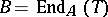. One can then assign tothe functors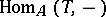,,, and, which are called tilting functors. The importance of considering such functors is that they give equivalences between subcategories of the module categories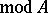and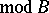, results first established by S. Brenner and M.C.R. Butler. Namely,and its adjointgive an equivalence between the subcategories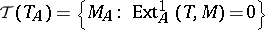and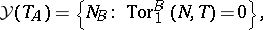while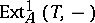andgive an equivalence between the subcategories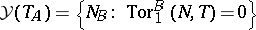and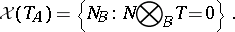It is not difficult to see thatand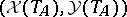are torsion pairs inand, respectively. Clearly, one can now transfer information fromto. One of the most interesting cases occurs whenis a hereditary algebra and so the torsion pairsplits, giving in particular that each indecomposable-module is the image of an indecomposable-module either byor by(in this case, the algebrais called tilted, cf. also Tilted algebra).

This procedure has been generalized in several ways and it is worthwhile mentioning, for instance, its connection with derived categories (cf. also Derived category), or the notion of quasi-tilted algebras. It has also been considered for infinitely-generated modules over arbitrary rings.

For referenes, see also Tilting theory; Tilted algebra.

How to Cite This Entry:
Tilting functor. FlÃ¡vio Ulhoa Coelho (originator), Encyclopedia of Mathematics. URL: http://www.encyclopediaofmath.org/index.php?title=Tilting_functor&oldid=13154
This text originally appeared in Encyclopedia of Mathematics - ISBN 1402006098